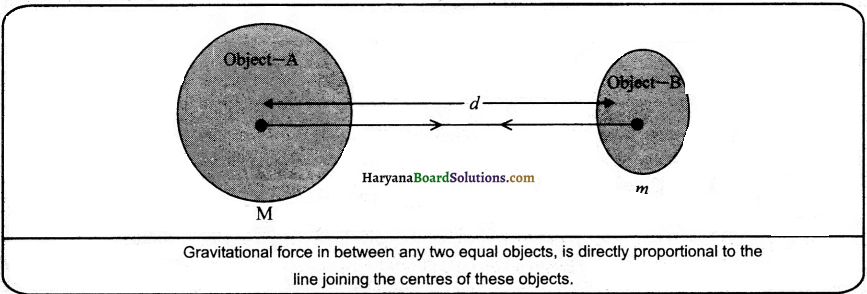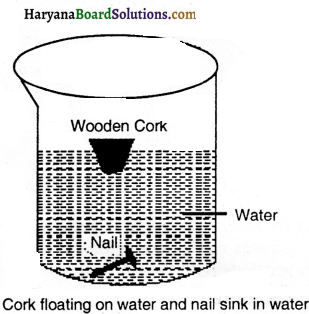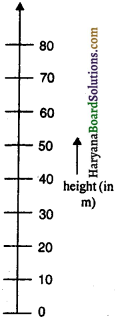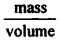# HBSE 9th Class Science Solutions Chapter 10 Gravitation

Haryana State Board HBSE 9th Class Science Solutions Chapter 10 Gravitation Textbook Exercise Questions and Answers.

## Haryana Board 9th Class Science Solutions Chapter 10 Gravitation

HBSE 9th Class Science Gravitation Intext Questions and Answers

Questions from Sub-section 10.1

Question 1.
State the universal law of gravitation.
Universal Law of Gravitation:
Every object in the universe attracts every other object with a force which is proportional to the product of their masses and inversely proportional to the square of thedistance between them. The force is along the line joining the centres of two objects. Let two objects A and B of masses M and ‘m lies at a distance ‘d’ from each other as shown in figure. Let the force of attraction between two objects be F. According to the universal law of gravitation, the force between two objects is directly proportional to the product of their masses, that is,
F ∝ M × m ……….(i)
And the force between two objects is inversely proportional to the square of the distance between them,
that is,
F ∝ $$\frac{1}{d^2}$$ ………..(ii)
Combining eqs. (i) and (ii), we get
F ∝ $$\frac{M \times m}{d^2}$$ or F = G $$\frac{M \times m}{d^2}$$
Where G is the constant of proportionality and is called universal gravitational constant.Question 2.
Write the formula to find the magnitude of the gravitational force between the earth and an object on the surface of the earth.
Suppose the mass of earth = Me
Mass of the object on the surface of earth = m
The radius of earth = R
∴ The magnitude of the gravitational force between the earth and an object on the surface of the earth (F) = G $$\frac{M_e \times m}{R^2}$$ (where G is gravitational constant)

Questions from Sub-section 10.2

Question 1.
What do you mean by free fall?
When the objects fall towards earth only because of gravitation, then it is said that the objects are in free faIl.

Question 2.
What do you mean by acceleration due to gravity?
When a body falls towards earth, acceleration works. This acceleration is due to the force of gravity of earth. Therefore, this acceleration is called the acceleration due to the force of gravity of earth or acceleration due to gravity. it is denoted by ‘g’ and its unit is ms2.Questions from Sub-sections 10.3, 10.4

Question 1.
What are the differences between the mass of an object and its weight?
The differences between the mass and weight of an object are as follows:

Mass:
1. Mass of a body is the quantity of matter contained in it.
2. The mass of an object cannot be zero.
3. Mass of an object is constant.
4. The mass of an object is found by physical balance.
5. Mass is measured ¡n kilogram (kg).
6. Mass is a scalar quantity.

Weight:
The force by which the earth attracts an object
towards itself is called the weight of that object on earth.
2. The weight of an object is zero on the centre of earth.
3. Weight is not constant. The weight of an object is more on poles as compared to equator.
4. The weight of an object is found by spring balance.
5. Weight is measured in newton.
6. Weight is a vector quantity.

Question 2.
Why is the weight of an object on the moon th its weight on the earth?
We know that the value of acceleration due to gravity (g) on earth is 9.8 ms-2. The value of g on moon is $$\frac {1}{6}$$ th time of that on earth. Therefore, the weight of objects on moon is less than that on earth. That is g $$\frac {1}{6}$$th times.

Questions from Sub-section 10.5

Question 1.
Why is it difficult to hold a school bag having a strap made of a thin and strong string?
It is difficult to hold a school bag having a strap made of a thin and strong string because its thrust affects a smaller area (in the center of the string).Question 2.
What do you mean by buoyancy?
When an object is immersed in a fluid, the fluid exerts a force on the body in an upward direction which is called buoyant force. The result of buoyant force depends upon the density of the fluid. This is known as buoyancy.

Question 3.
Why does an object float or sink when placed on the surface of the water?
Objects of density less than that of a liquid float on the liquid. The objects of density greater than that of a liquid sink in the liquid. For example, when a nail and a cork of the same mass are put in water, the nail will sink because its density is more than water, whereas the cork will float because its density is less than that of water.Questions from Sub-section 10.6

Question 1.
You find your mass to be 42 kg on a weighing machine. Is your mass more or less than 42 kg?
if the mass is 42 kg on a weighing machine, then our mass will be 42 kg because mass remains constant.

Question 2.
You have a bag of cotton and an iron bar, each indicating a mass of 100 kg when measured on a weighing machine. In reality, one is heavier than other. Can you say which one ¡s heavier and why?
Iron bar will be heavier than a bag of cotton because the density of iron is more than cotton.

HBSE 9th Class Science Gravitation Textbook Questions and Answers

Question 1.
How does the force of gravitation between two objects change when the distance between them is reduced to half?
If the distance between two objects is reduced to half, then the gravitational force between them will increase 4 times, because gravitational force is inversely proportional to the square of the distance between them.Question 2.
Gravitational force acts on all objects is proportional to their masses. Why then, a heavy object does not fall faster than a light object?
Gravitational force acts on all objects is proportional to their masses, yet a heavy object will not fall faster than a light object because the mass of objects is negligible in comparison to that of earth.

Question 3.
What is the magnitude of the gravitational force between the earth and a 1 kg object on its surface? (Mass of the earth is 6 x 1024 kg and radius of the earth is 6.4 x 106m.)
Solution:
Here, Mass of earth (Me) = 6 × 1024kg
Mass of object (m) = 1 kg
Radius of earth (R) = 6.4 × 106m
Gravitational constant (G) = 6.673 × 101 Nm2 kg2
Force of gravity (g) = ?
We know that,
Force of gravity (g) = $$\frac{G M_e \times m}{R^2}$$ = $$\frac{6.673 \times 10^{-11} \times 6 \times 10^{24} \times 1}{\left(6.4 \times 10^6\right)^2}$$ = 9.8N

Question 4.
The earth and the moon are attracted to each other by gravitational force. Does the earth attract the moon with a force that is greater or smaller or the same as the force with which the moon attracts the earth? Why?
The earth attracts the moon with greater force because the mass of earth is more than that of moon.

Question 5.
If the moon attracts the earth, why does the earth not move towards the moon?
The moon attracts the earth, but the earth does not move towards the moon because the mass of the moon is much less than that of earth.

Question 6.
What happens to the force between two objects, if:
(i) the mass of one object is doubled?
(ii) the distance between the objects is doubled and tripled?
(iii) the masses of both objects are doubled?
(i) If the mass of one object is doubled, then the force between two objects will be doubled because gravitational force is proportional to mass.
(ii) If the distance between two objects is doubled, the gravitational force will become times and if the distance is tripled, the gravitational force will become times, because gravitational force is inversely proportional to the square of the distance.
(iii) As the force between two bodies is proportional to the product of masses of the bodies, so if the masses of both the bodies are doubled, then force between them will become four times.Question 7.
What i.s the importance of universal law of gravitation?
The importance of universal law of gravitation is as follows:
(i) This force binds us to the earth.
(ii) The motion of the moon around the earth is due to this force.
(iii) The motion of planets around the sun is due to this force.
(iv) The tides due to the moon and the sun are due to this force.

Question 8.
What is the acceleration of free fall?
When an object falls towards the earth, then acceleration works. Acceleration of free fall is, acceleration due to the gravitational force of earth and is equal to g. Its value is 9.8 ms2.

Question 9.
What do we cafl the gravitational force between the earth and an object?
The gravitational force between the earth and an object is weight.

Question 10.
Amit buys few grams of gold at the poles as per the Instruction of one of his friends. He hands over the same when he meets him at the equator. Will the friend agree with the weight of gold bought? If not, why ? (Hint: The value of g is greater at the poles than at the equator.)
No, the friend will not agree with the weight of gold bought, because the weight of gold is less on the equator than on the poles.

Question 11.
Why will a sheet of paper fall slower than one of that ¡s crumpled into a ball?
The sheet of paper will fall slower than one of that is crumpled into a ball because the area of sheet is more than the area of ball, due to this, the pressure decreases.Question 12.
The gravitational force on the surface of the moon is only as strong as gravitational force on the earth. What is the weight In newtons of a 10 kg object on the moon and on the earth?
Solution:
Here,
Mass of the object on earth (m) = 10kg
Acceleration due to gravity on earth (g) = 9.8 m/s2
Weight of the object on earth (w) m x g = 10 x 9.8 N = 98 N
Mass of object on moon (m) = 10 kg
Acceleration due to gravity on moon (g1) = $$\frac {9.8}{6}$$m/s2
∴ Weight of the object on moon (w) = mg1 = 10 x $$\frac {9.8}{6}$$ N = 16.3 N

Question 13.
A ball ¡s thrown vertically upwards with a velocity 49 m’s. Calculate:
(i) the maximum height to which it rises.
(ii) the total time it takes to return to the surface of the earth.
Solution:
Here,
Initial velocity of ball (u) = 49 m/s
Final velocity of ball (v) = 0 m/s
Acceleration due to gravity (g) = -9.8 m/s2
(i) We know that,
v2 – u2 = 2gs
s = $$\frac{v^2-u^2}{2 g}=\frac{(0)^2-(49)^2}{2(-9.8)}$$ = $$\frac{-49 \times 49}{-19.6}$$ = 122.5m
∴ The maximum height to which ball rises is 122.5 m

(ii) Time taken (i) = $$\frac{v-u}{g}$$ = $$\frac{0-49}{-9.8}$$ = 5s
∴ The total time it takes to return to the surface of earth 5s + 5s = 10

Question 14.
A stone is released from the top of a tower of a height of 19.6 m. Calculate Its final velocity just before touching the ground.
Solution:
Here,
Initial velocity of stone (u) = 0 m/s
The final velocity of stone (v) =?
Height of the top of the tower (s) = 19.6 m
Acceleration due to gravity (g) = 9.8 ms-2
We know that,
v2 – u2= 2gs
v2 – (0)2 = 2 x 9.8 x 19.6
or v2 = 19.6 x 19.6
or v = $$\sqrt{19.6 \times 19.6}$$= 19.6 ms-1
∴ Thus velocity of stone will be 19.6 ms-1.Question 15.
A stone is thrown vertically upward with an initial velocity of 40 ms. Taking g = 10 m/s2, find the maximum height reached by the stone. What is the net displacement and the total distance covered by the stone?
Solution:
Here,
Initial velocity of stone (u) = 40 m/s
Final velocity of stone (v) = 0 m/s
Acceleration due to gravity (s) = -10 m/s2We know that,
v2 – u2 = 2gs
s = $$\frac{v^2-u^2}{2 g}=\frac{(0)^2-(40)^2}{2(-10)}$$ = $$\frac{-40 \times 40}{-2 \times 10}$$ = 80m
∴ The maximum height reached by the stone = 80 m
Total distance covered by the stone = 80 m + 80 m = 160m
TotaÌ displacement of the stone = ZeroQuestion 16.
Calculate the foíce of gravitation between the earth and the Sun, given that the mass of the earth = 6 x 1024 kg and of the Sun 2 x 1030 kg. The average distance between the two Is 1.5 x 1011 m.
Solution:
Here, The mass of the earth (Me) = 6 x 1024 kg
Mass of the sun (Me) = 2 x 1030 kg
The average distance between the two (Rs) = 1.5 x 1011 m
Gravitational constant (G) = 6.673 x 1011 Nm2/kg2
∴ Force of gravitation between the earth and sun (Fs) = $$\frac{G M_e M_s}{\left(R_s\right)^2}$$
= $$\frac{6.673 \times 10^{-11} \times 6 \times 10^{24} \times 2 \times 10^{30}}{\left(1.5 \times 10^{11}\right)^2}$$ N
= $$\frac{6.673 \times 12 \times 10^{43}}{2.25 \times 10^{22}}$$ N = 35.59 x 1021 N

Question 17.
A stone is allowed to fall from the top of a tower 100 m high and at the same time another stone is projected vertically upwards from the ground with a velocity of 25 m/s. Calculate when and where the two stones will meet.
Solution:
Suppose the two stones will meet at a distance of m.
For the stone tell from the top;
initial velocity (u) = 0 m/s
Acceleration due to gravity (g) = 9.8 m/s2
Distance covered (s) = x m
We know that
S = ut + $$\frac {1}{2}$$ gt2
x = (0) (t) + $$\frac {1}{2}$$ (9.8)t2
or x = 4.9 t2 ………….(i)

For the stone projected upwards;
Initial velocity (u) = 25 m/s
Acceleration due to gravity (g) = -9.8 ms2
Distance covered (s) = (100 – x)m
We know that,
(s) = ut+ $$\frac {1}{2}$$ gt2
100 – x 251 + $$\frac {1}{2}$$ (-9.8) t2
or 100 – 4.9t2 = 25 t – 4.9 t2 [From (i)]
or 100 = 25 t
or t = $$\frac {100}{25}$$ = 4s

Both stones will meet after 4 seconds Ans
Height from top (x) = 4.9 (4)2
4.9 x 16 = 78.4 m
∴ Distance from down = 100 – 78.4 = 21.6 mQuestion 18.
A ball thrown up vertically returns to the thrower after 6 s. Find
(a) the velocity with which it was thrown up.
(b) the maximum height It reaches and
(c) its position after 4s.
Solution:
Here,
Initial velocity (u) = ?
Final velocity (v) = 0 m/s
Time taken by ball to cover maximum height (t) = $$\frac {6s}{2}$$ = 3s
Upward acceleration due to gravity (g) = – 9.8 m/s2
(a) We know that
v = u + gt
u = v – gt = 0 – (-.9.8)(3) = 29.4 m/s
Therefore, initial velocity of ball (u) = 29.4 m/s

(b) We know that
s = ut + $$\frac {1}{2}$$ gt2
= (29.4) (3) + $$\frac {1}{2}$$ (-9.8) (3)2
88.2 – 44.1 = 44.1 m
∴ Maximum height it reaches = 44.1 m

(c) For this state, distance will be found from up to down
u = 0
t = 4s – 3s = 1s
g = 9.8 m/s2
s = ut + $$\frac {1}{2}$$ gt2
0(1) + $$\frac {1}{2}$$ (9.8)(1)2
= 4.9m
∴ The ball will be at a height of 4.9 m from up.
Height of ball from down = 44.1 – 4.9 = 39.2 m

Question 19.
In what direction does the buoyant force on an object Immersed in a liquid act?
The buoyant force on an object immersed in a liquid acts in the vertically upward direction.

Question 20.
Why does a block of plastic released under water come up to the surface of the water?
The block of plastic when left under water comes to the surface because the thrust due to water is greater than the weight of the block itself.Question 21.
The volume of 50 g of. the substance is 20. If the density of water is 1 gcm4, will the substance float or sink?
Solution:
Here,
Mass of the substance = 50g
Volume of the substance = 20 cm3
∴Density of substance == $$\frac {50}{20}$$ g cm-3
= 2.5g cm-3
Since the density of the substance is greater than that of water, hence, the substance will sink in water.

Question 22.
The volume of. 500 g sealed packet is 350 cm3. Will the packet float or sink in water if the density of water Is 1g cm3? What will be the mass of the water displaced by this packet?
Solution:
Here,
Mass of the packet = 500g
Volume of the packet = 350 cm3
Density of packet == $$\frac {500}{350}$$ g cm-3 = 1.43 gcm-3
Since the density of packet is greater than that of water, hence, it will sink in water.
Mass of water displaced = volume x density = 350 x 1 g = 350 g